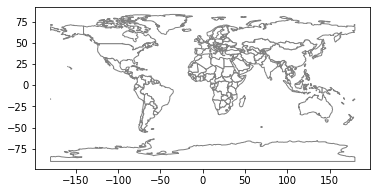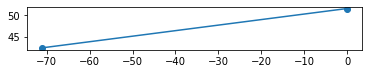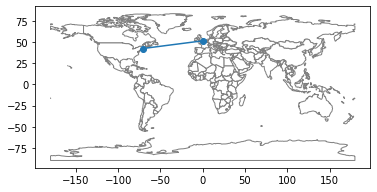# Times and places¶

In the previous chapter, you learned about variables and two kinds of values: integers and floating-point numbers.

In this chapter, you’ll see some additional types:

• Strings, which represent text.

• Time stamps, which represent dates and times.

• And several ways to represent and display geographical locations.

Not every data science project uses all of these types, but many projects use at least one.

## Strings¶

A string is a sequence of letters, numbers, and punctuation marks. In Python you can create a string by typing letters between single or double quotation marks.

'Elements'

'Elements'

"of"

'of'


And you can assign string values to variables.

first = 'Data'

last = "Science"


Some arithmetic operators work with strings, but they might no do what you expect. For example, the + operator “concatenates” two strings; that is, it creates a new string that contains the first string followed by the second string:

first + last

'DataScience'


If you want to put a space between the words, you can use a string that contains a space:

first + ' ' + last

'Data Science'


Strings are used to store text data like names, addresses, titles, etc.

When you read data from a file, you might see values that look like numbers, but they are actually strings, like this:

not_actually_a_number = '123'


If you try to do math with these strings, you might get an error. For example, the following expression causes a TypeError with the message “can only concatenate str (not int) to str”.

not_actually_a_number + 1


But you don’t always get an error; instead, you might get a surprising result. For example:

not_actually_a_number * 3

'123123123'


If you multiply a string by an integer, Python repeats the string the given number of times.

If you have a string that contains only digits, you can convert it to an integer using the int function:

int('123')

123


Or you can convert it to a floating-point number using float:

float('123')

123.0


But if the string contains a decimal point, you can’t convert it to an int.

Going in the other direction, you can convert any type of value to a string using str:

str(123)

'123'

str(12.3)

'12.3'


Exercise: When personal names are stored in a database, they are often stored in three variables: a given name, a family name, and sometimes a middle name. For example, a list of great rock drummers might include:

given = 'Neil'
middle = 'Ellwood'
family = 'Peart'


But names are often displayed different ways in different contexts. For example, the first time you mention someone in an article, you might give all three names, like “Neil Ellwood Peart”. But in the index of a book, you might put the family name first, like “Peart, Neil Ellwood”.

Write Python expressions that use the variables given, middle, and family to display Neil Peart’s name in these two formats.

## Dates and times¶

If you read data from a file, you might also find that dates and times are represented with strings.

not_really_a_date = 'June 4, 1989'


To confirm that this value is a string, we can use the type function, which takes a value and reports its type.

type(not_really_a_date)

str


str indicates that the value of not_really_a_date is a string.

We get the same result with not_really_a_time, below:

not_really_a_time = '6:30:00'
type(not_really_a_time)

str


Strings that represent dates and times a readable for people, but they are not useful for computation.

Fortunately, Python provides libraries for working with date and time data; the one we’ll use is called Pandas. As always, we have to import a library before we use it; it is conventional to import Pandas with the abbreviated name pd:

import pandas as pd


Pandas provides a type called Timestamp, which represents a date and time.

It also provides a function called Timestamp, which we can use to convert a string to a Timestamp:

pd.Timestamp('6:30:00')

Timestamp('2021-02-23 06:30:00')


Or we can do the same thing using the variable defined above.

pd.Timestamp(not_really_a_time)

Timestamp('2021-02-23 06:30:00')


In this example, the string specifies a time but no date, so Pandas fills in today’s date.

A Timestamp is a value, so you can assign it to a variable.

date_of_birth = pd.Timestamp('June 4, 1989')
date_of_birth

Timestamp('1989-06-04 00:00:00')


If the string specifies a date but no time, Pandas fills in midnight as the default time.

If you assign the Timestamp to a variable, you can use the variable name to get the year, month, and day, like this:

date_of_birth.year, date_of_birth.month, date_of_birth.day

(1989, 6, 4)


You can also get the name of the month and the day of the week.

date_of_birth.day_name(), date_of_birth.month_name()

('Sunday', 'June')


Timestamp provides a function called now that returns the current date and time.

now = pd.Timestamp.now()
now

Timestamp('2021-02-23 11:59:43.399056')


Exercise: Use the value of now to display the name of the current month and day of the week.

## Timedelta¶

Timestamp values support some arithmetic operations. For example, you can compute the difference between two Timestamps:

age = now - date_of_birth
age

Timedelta('11587 days 11:59:43.399056')


The result is a Timedelta that represents the current age of someone born on date_of_birth. The Timedelta contains components that store the number of days, hours, etc. between the two Timestamp values.

age.components

Components(days=11587, hours=11, minutes=59, seconds=43, milliseconds=399, microseconds=56, nanoseconds=0)


You can get one of the components like this:

age.days

11587


The biggest component of Timedelta is days, not years, because days are well defined and years are problematic.

Most years are 365 days, but some are 366. The average calendar year is 365.24 days, which is a very good approximation of a solar year, but it is not exact (see https://pumas.jpl.nasa.gov/files/04_21_97_1.pdf).

One way to compute age in years is to divide age in days by 365.24:

age.days / 365.24

31.724345635746356


But people usually report their ages in integer years. We can use the Numpy floor function to round down:

import numpy as np

np.floor(age.days / 365.24)

31.0


Or the ceil function (which stands for “ceiling”) to round up:

np.ceil(age.days / 365.24)

32.0


We can also compare Timestamp values to see which comes first. For example, let’s see if a person with a given birthdate has already had a birthday this year. Here’s a new Timestamp with the year from now and the month and day from date_of_birth.

bday_this_year = pd.Timestamp(now.year,
date_of_birth.month,
date_of_birth.day)
bday_this_year

Timestamp('2021-06-04 00:00:00')


The result represents the person’s birthday this year. Now we can use the > operator to check whether now is later than the birthday:

now > bday_this_year

False


The result is either True or False. These values belong to a type called bool, short for “Boolean algebra”, which is a branch of algebra where all values are either true or false.

type(True)

bool

type(False)

bool


Exercise: Any two people with different birthdays have a “Double Day” when one is twice as old as the other. Suppose you are given two Timestamp values, d1 and d2, that represent birthdays for two people. Use Timestamp arithmetic to compute their double day. With the following dates, the result should be December 19, 2009.

d1 = pd.Timestamp('2003-07-12')
d2 = pd.Timestamp('2006-09-30')


## Location¶

There are many ways to represent geographical locations, but the most common, at least for global data, is latitude and longitude.

When stored as strings, latitude and longitude are expressed in degrees with compass directions N, S, E, and W. For example, this string represents the location of Boston, MA, USA:

lat_lon_string = '42.3601° N, 71.0589° W'


When we compute with location information, we use floating-point numbers, with

• Positive latitude for the northern hemisphere, negative latitude for the southern hemisphere, and

• Positive longitude for the eastern hemisphere and negative longitude for the western hemisphere.

Of course, the choice of the origin and the orientation of positive and negative are arbitrary choices that were made for historical reasons. We might not be able to change conventions like these, but we should be aware that they are conventions.

Here’s how we might represent the location of Boston with two variables.

lat = 42.3601
lon = -71.0589


It is also possible to combine two numbers into a composite value and assign it to a single variable:

boston = lat, lon
boston

(42.3601, -71.0589)


The type of this variable is tuple, which is a mathematical term for a value that contains a sequence of elements. Math people pronounce it “tuh’ ple”, but computational people usually say “too’ ple”. Take your pick.

type(boston)

tuple


If you have a tuple with two elements, you can assign them to two variables, like this:

y, x = boston
y

42.3601

x

-71.0589


Notice that I assigned latitude to y and longitude to x, because a y coordinate usually goes up and down like latitude, and an x coordinate usually goes side-to-side like longitude.

Exercise: Find the latitude and longitude of the place you were born or someplace you think of as your “home town”. You can use this web page to look it up. Make a tuple of floating-point numbers that represents that location.

## Distance¶

If you are given two tuples that represent locations, you can compute the approximate distance between them, along the surface of the globe, using the haversine function. If you are curious about it, you can read an explanation in this article. To estimate a haversine distance, we have to compute the haversine function, which is defined:

$$\mathrm{haversine}(\theta)=\sin^2(\theta/2)$$

Where $$\theta$$ is an angle in radians. We can compute this function in Python like this:

import numpy as np

θ = 1
np.sin(θ/2)**2

0.22984884706593015


You can use Greek letters in variable names, but there is currently no way to type them in Jupyter/Colab, so I usually copy them from a web page and paste them in.

To avoid the inconvenience, it is more common to write out letter names, like this:

theta = 1
np.sin(theta/2)**2

0.22984884706593015


Remember that the operator for exponentiation is **.
In some other languages it’s ^, which is also an operator in Python, but it performs another operation altogether.

## Defining functions¶

At this point you don’t have to know how to define a new function. But you will see function definitions, so I want to explain the basics now.

If we are planning to use an expression like np.sin(theta/2)**2 more than a few times, we can define a new function that computes it, like this:

def haversine(theta):
"""Compute the haversine function of theta."""
return np.sin(theta/2)**2


On the first line, def indicates that we are defining a function.

The second line is a “triple-quoted string”, which describes what the function does, but it has no effect when the program runs.

On the third line, return indicates the result of the function.

When you run the previous cell, it creates a new variable called haversine. You can display its value like this:

haversine

<function __main__.haversine(theta)>


And you can display its type like this:

type(haversine)

function


So haversine is a variable that refers to a function. To run the function and compute a result, we have to “call” the function and provide a value for theta:

haversine(1)

0.22984884706593015


When you define a function, you create a new variable. But the function doesn’t actually run until you call it.

## Haversine distance¶

Now we can use haversine as part of a function that computes haversine distances. I won’t explain this function in as much detail, but if you read through it, you might get a sense of how it works.

def haversine_distance(coord1, coord2):
"""Haversine distance between two locations.

coord1: lat-lon as tuple of float
coord2: lat-lon as tuple of float

returns: distance in km
"""
R = 6372.8  # Earth radius in km
lat1, lon1 = coord1
lat2, lon2 = coord2

a = haversine(dphi) + np.cos(phi1)*np.cos(phi2)*haversine(dlambda)

distance = 2*R*np.arctan2(np.sqrt(a), np.sqrt(1 - a))

return distance


When we call this function, we provide two tuples, each representing a latitude and a longitude. We already have a tuple that represents the location of Boston. Now here’s a tuple that represents the location of London, England, UK:

london = 51.5074, -0.1278


And here’s the haversine distance between Boston and London.

haversine_distance(boston, london)

5265.656325981015


The actual geographic distance is slightly different because Earth is not a perfect sphere. But the error of this estimate is less than 1%.

Exercise: Use haversine_distance to compute the distance between Boston and your home town from the previous exercise.

If possible, use an online map to check the result.

## Geopandas¶

Python provides libraries for working with geographical data. One of the most popular is Geopandas, which is based on another library called Shapely. Shapely provides Point and LineString values, which we’ll use to represent geographic locations and lines between them.

from shapely.geometry import Point, LineString


We can use the tuples we defined in the previous section to create Shapely Point values, but we have to reverse the order of the coordinates, providing them in $$x$$-$$y$$ order rather than lat-lon order, because that’s the order the Point function expects.

lat, lon = boston
p1 = Point(lon, lat)

lat, lon = london
p2 = Point(lon, lat)


We can use the points we just defined to create a LineString:

line = LineString([p1, p2])


Now we can use Geopandas to show these points and lines on a map. The following code loads a map of the world and plots it.

import geopandas as gpd

path = gpd.datasets.get_path('naturalearth_lowres')
world.plot(color='white', edgecolor='gray');


By default, Geopandas uses an equirectangular projection, which provides a misleading picture of relative land areas (see https://en.wikipedia.org/wiki/Equirectangular_projection). You can’t make a map without making visualization decisions.

Now let’s put dots on the map for Boston and London. We have to put the Point values and the LineString into a GeoSeries.

t = [p1, p2, line]
series = gpd.GeoSeries(t)


Here’s a first attempt to plot the maps and the lines together:

# plot the map
world.plot(color='white', edgecolor='gray')

# plot Boston, London, and the line
series.plot();The two plots are on different axes, which is not what we want in this case.

To get the points and the map on the same axes, we have to use a function from Matplotlib, which is a visualization library we will use extensively. We’ll import it like this.

import matplotlib.pyplot as plt


The function is gca, which stands for “get current axes”. We can use the result to tell plot to put the points and lines on the current axes, rather than create a new one.

ax = plt.gca()
world.plot(color='white', edgecolor='gray', ax=ax)
series.plot(ax=ax);There are a few features in this example I have not explained completely, but hopefully you get the idea.

Exercise: Modify the code in this section to plot a point that shows the home town you chose in a previous exercise and a line from there to Boston.

Then go to this online survey and answer the questions there.